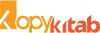# DISCRETE PROBABILITY AND PROBABILITY DISTRIBUTIONS 2 : STATISTICS (ST-122) : PAPER II (2)

75.00DISCRETE PROBABILITY AND PROBABILITY DISTRIBUTIONS 2 : STATISTICS (ST-122) : PAPER II (2)

75.00

 Authors Name Dr. (Mrs.) V. R. Prayag , Dr. P. G. Dixit , P. S. Kapse ISBN 13 9789389533637 Publisher Nirali Prakashan Edition First Buy E-Book (PDF) [Click on Logo >>]Pages 140 Language English Publishing Year Oct-19
For E-Books purchased from Kopykitab.com For E-Books purchased from Amazon
##### Description

1. Some Standard Discrete Probability Distribution Countably Infinite Sample Space,
2. Bivariate Discrete Probability Distributions,
3. Mathematical Expectation (Bivariate),
* Appendix A,
* Appendix B,
* Appendix C,
Model Question Paper

We feel indeed very happy to present this text-book of ‘Statistics Paper-II’ – ‘Discrete Probability and Probability Distributions-II’ to the students of F.Y.B.Sc. and F.Y.B.A. The book is written according to the revised syllabus of CBCS Pattern of Savitribai Phule Pune University with effective from June 2019.

The main purpose of the book is to provide foundation as well as comprehensive background of ‘Discrete Probability Theory’ to beginners in simple and interesting manner. In order to make the contents of the book easier to comprehend, we have included a requisite number of illustrations, remarks, figures, diagrams etc. to elucidate statistical concepts. Application of Statistics in real life situations is emphasized through illustrative examples. Ample number of graded problems, theoretical as well as numerical, are provided at the end of each chapter along with hints and answers. The numerical problems will also be useful for the F.Y.B.Sc. students to prepare for Paper – III : Practicals. A list of practicals is given in the syllabus. Appendix A compiles some important mathematical results which are needed during the entire course. Values of individual terms of binomial probabilities are given in Appendix B. A specimen paper is set for student’s self assessment.